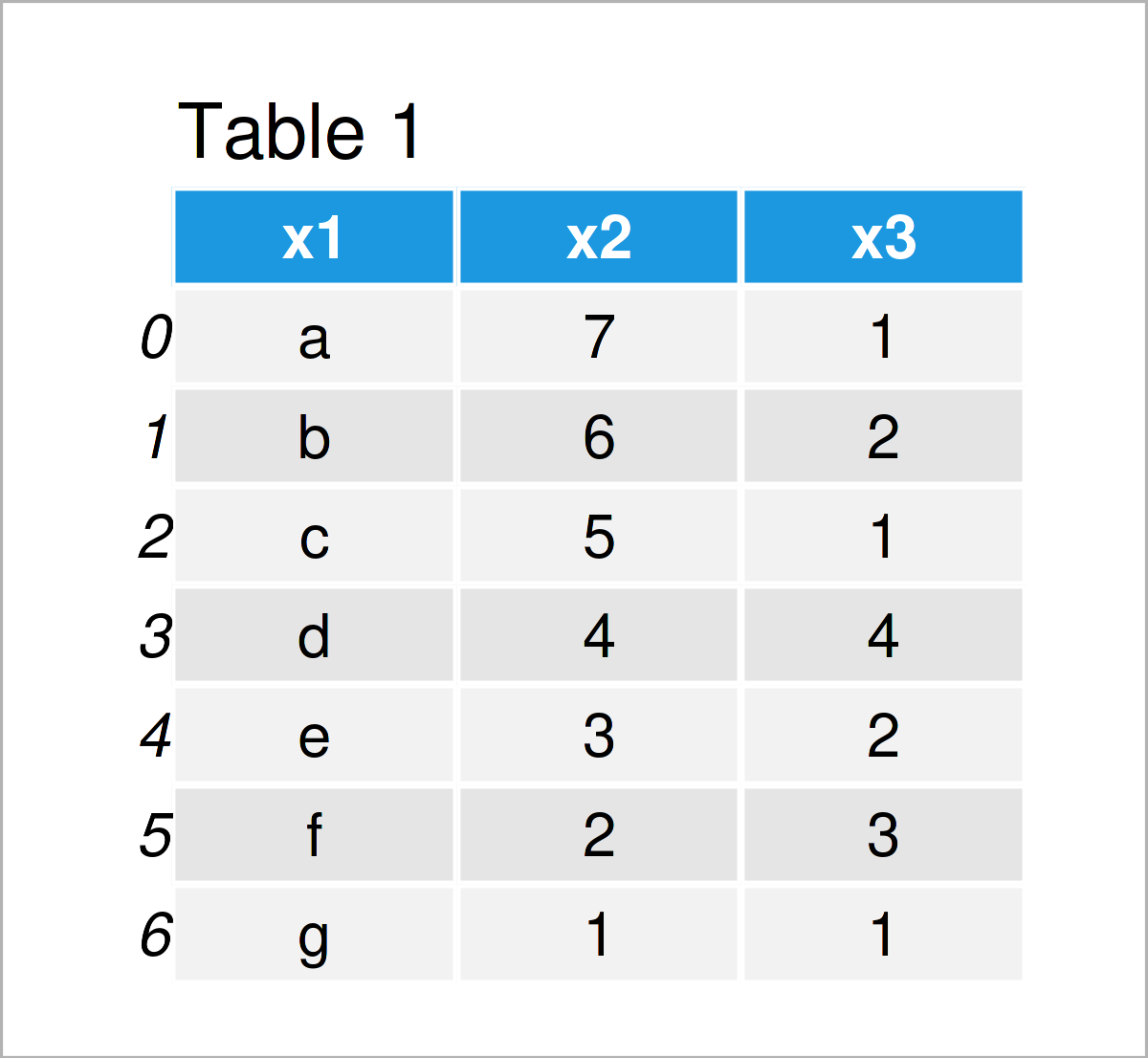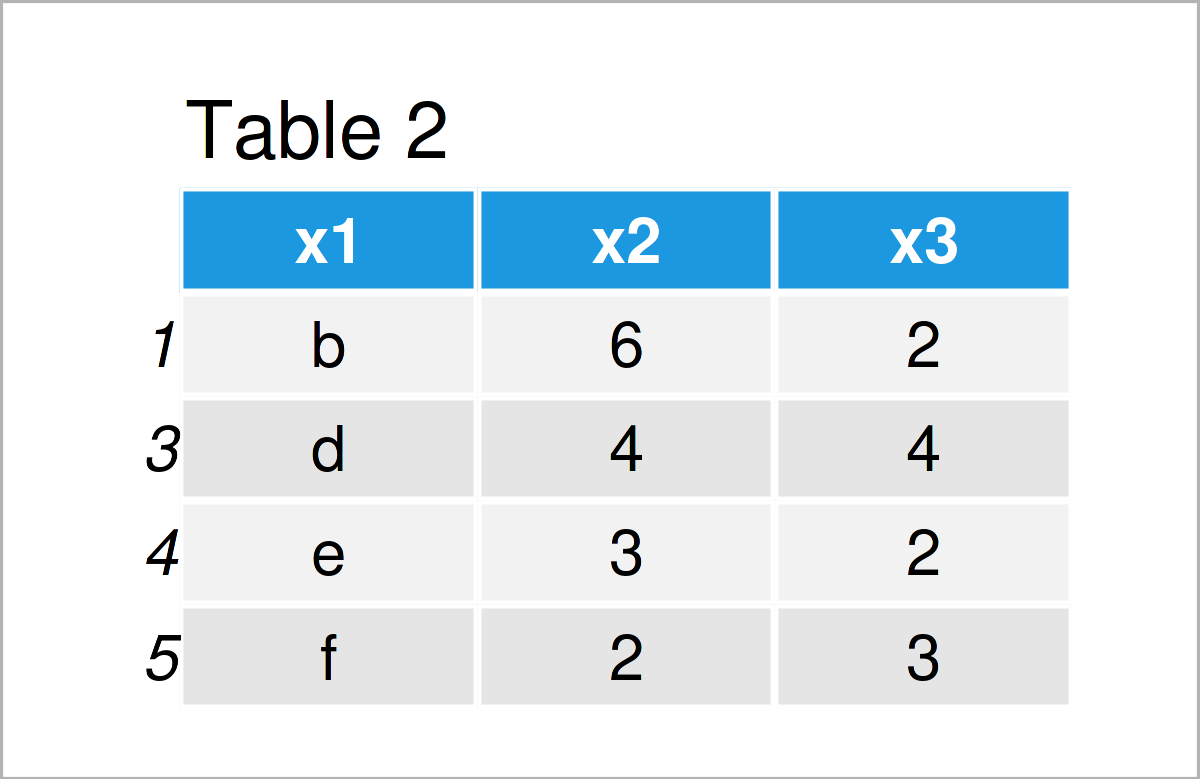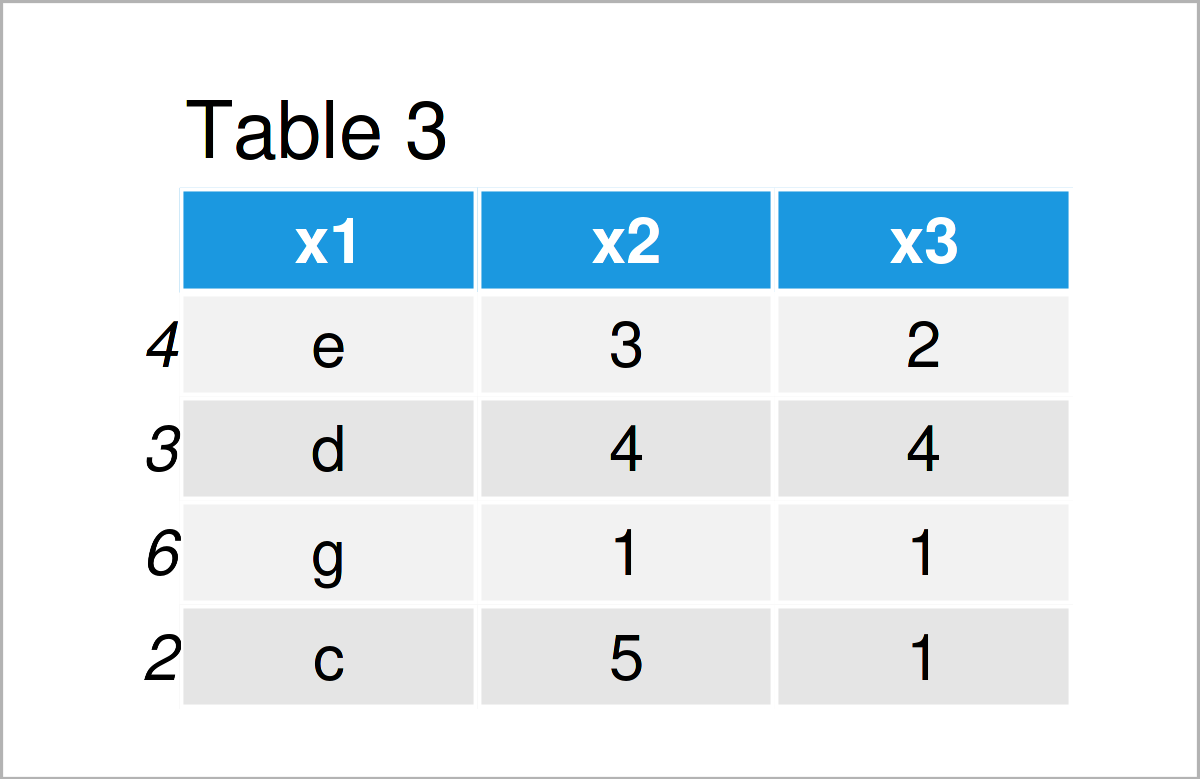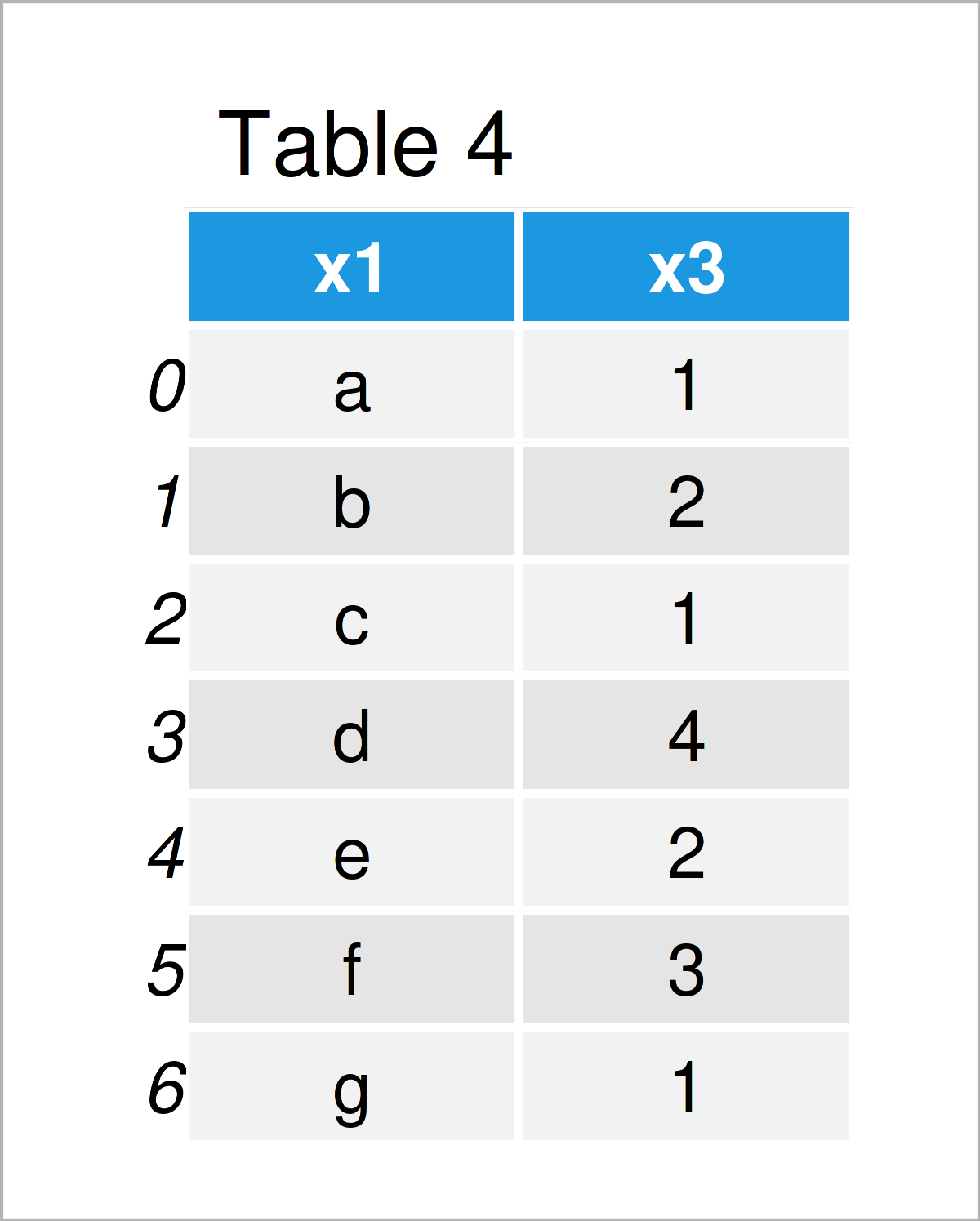# Create Subset of pandas DataFrame in Python (3 Examples)

In this Python programming article you’ll learn how to subset the rows and columns of a pandas DataFrame.

The post is structured as follows:

Let’s take a look at some Python codes in action!

## Example Data & Libraries

First, we need to import the pandas library:

`import pandas as pd                                             # Import pandas library in Python`

In addition, have a look at the following example data:

```data = pd.DataFrame({'x1':['a', 'b', 'c', 'd', 'e', 'f', 'g'],  # Create pandas DataFrame
'x2':range(7, 0, - 1),
'x3':[1, 2, 1, 4, 2, 3, 1]})
print(data)                                                     # Print pandas DataFrame```Have a look at the table that has been returned after running the previous Python code. It shows that our exemplifying pandas DataFrame contains seven rows and three columns.

## Example 1: Create pandas DataFrame Subset Based on Logical Condition

Example 1 shows how to subset the rows of a pandas DataFrame conditionally.

For this task, we have to specify a logical condition for a column within the loc attribute:

```data_range = data.loc[data['x3'] >= 2]                          # Get rows in range
print(data_range)                                               # Print DataFrame subset```In Table 2 you can see that we have created a new pandas DataFrame consisting of a subset of rows of our input DataFrame.

## Example 2: Randomly Sample pandas DataFrame Subset

Example 2 demonstrates how to generate a random subsample of a pandas DataFrame.

For this task, we need the functions of the NumPy library. In order to use the functions of the NumPy library, we need to import NumPy:

`import numpy                                                    # Import numpy`

In the next step, we can use the numpy.random.seed function to make our example reproducible:

`numpy.random.seed(436862)                                       # Set random seed`

Now, we can apply the sample function to randomly select a certain fraction of the rows in our pandas DataFrame:

```data_sample = data.sample(frac = 0.5)                           # Draw random subset
print(data_sample)                                              # Print DataFrame subset```Table 3 shows the output of the previous code: A random subsample of our input data set.

## Example 3: Create Subset of Columns in pandas DataFrame

This example illustrates how to create a subset of the columns of a pandas DataFrame in Python programming.

To achieve this, we can use the syntax as shown below:

```data_cols = data[['x1', 'x3']]                                  # Extract certain columns
print(data_cols)                                                # Print DataFrame subset```By running the previous Python programming code, we have created Table 4, i.e. a pandas DataFrame containing only two of the three variables of our original data set.

## Video, Further Resources & Summary

Some time ago, I have published a video on my YouTube channel, which demonstrates the Python programming codes of this article. You can find the video below:

Furthermore, you might want to read some other articles on this homepage.